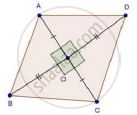# Each Side of a Rhombus is 10 Cm. If One of Its Diagonals is 16 Cm Find the Length of the Other Diagonal. - Mathematics

Each side of a rhombus is 10 cm. If one of its diagonals is 16 cm find the length of the other diagonal.

#### SolutionWe have,

ABCD is a rhombus with side 10 cm and diagonal BD = 16 cm

We know that diagonals of a rhombus bisect each other at 90°

∴ BO = OD = 8 cm

In ΔAOB, by pythagoras theorem

AO2 + BO2 = AB2

⇒ AO2 + 82 = 102

⇒ AO2 = 100 − 64 = 36

⇒ AO = sqrt36 = 6 cm          [By above property]

Hence, AC = 6 + 6 = 12 cm

Concept: Application of Pythagoras Theorem in Acute Angle and Obtuse Angle
Is there an error in this question or solution?

#### APPEARS IN

RD Sharma Class 10 Maths
Chapter 7 Triangles
Exercise 7.7 | Q 15 | Page 120